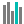#CHISQ.DIST (PQL - xl)

This function returns the criterion binomial, the smallest value for which the cumulative binomial distribution is greater than or equal to a criterion value

• Library: PQL \ Spreadsheet \ Statistical
• Compatibility: Any content (regardless of data source) in the Tabulate spreadsheet module
• Solve: This function can be used with the Solve plug-in: both as part of the objective function and constraint functions

#### Syntax

CHISQ.DIST(x, deg freedom, cumulative)

##### Function Arguments
 Name Description Type Optional x Value at which to evaluate the function Number deg freedom Number of degrees of freedom; if not an integer, the number is truncated Number cumulative A logical value that determines the form of the function. If cumulative is TRUE, this function returns the cumulative distribution function; if FALSE, it returns the probability density function StringOrNumber